×#### Thank you for registering.

One of our academic counsellors will contact you within 1 working day.

Click to Chat

1800-1023-196

+91-120-4616500

CART 0

• 0

MY CART (5)

Use Coupon: CART20 and get 20% off on all online Study Material

ITEM
DETAILS
MRP
DISCOUNT
FINAL PRICE
Total Price: Rs.

There are no items in this cart.
Continue Shopping• Complete JEE Main/Advanced Course and Test Series
• OFFERED PRICE: Rs. 15,900
• View Details

```Revision Notes on Comparing Quantities

Ratios

It shows the relation between two quantities or to compare two quantities.

Example

If there are 20 men and 40 women in a company then the ratio of the number of men to the number of women is 20: 40.

By using the fraction, it can be written as 20/40 = 1/2.

This shows that the number of men is half the number of women in the company.

It is written as 1: 2 and read as “1 is to 2”.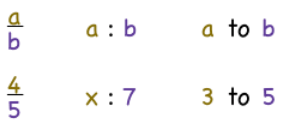Percentage

This is another way to compare the quantities. It means for every hundred. In the fraction form if the denominator is 100 then the numerator is the percentage and is represented by a special symbol %, read as a percent.

There are two methods to find the percentage.

1. By Fraction Method

Example

If there are 20 boys out of 50 students in the class then find the percentage of boys.

To make the denominator hundred we need to multiply both the denominator and numerator with 2.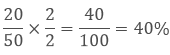So the percentage of boys is 40%.

2. Unitary Method

In the unitary method, first, we need to find the value of one unit then multiply it with the required number of units.

Example

In the above example, there are 20 boys out of 50 students. Then out of 100 students, the number of boys will beFinding the Increase or Decrease Percent

To find the increase or decrease in percent there are two methods.

1. Calculate the percentage of the given value then add or subtract it to the original value.

Example

If the price of the refrigerator was 50000 Rs. last year and it increases by 20 % this year. Then what is its price now?

Solution

First, we will calculate the 20% of 50000 Rs.Current Value = Original Value + Increased Price

= 50000 + 10000

= 60000 Rs.

The current value of the refrigerator is 60000 Rs.

2. Unitary Method

As we know that 20% increase means 100 Rs. will increase to 120 Rs.

So 50000 will increase toHence the increased value of the refrigerator has come directly.

Finding Discounts

It’s very common these days that there is a sale and the products are at a discounted price.

Basically discount is the reduction in the Market price to increase the sale and to promote the products.

We can get the amount of discount by subtracting the Sale price from the Market price.

Discount = Market price – Sale Price

To calculate the discount percentage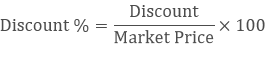Example

If the price of one jean is 2500 Rs. and we are getting a discount of 500 Rs. then what is the sale price of the jeans? What is the discount percentage?

Solution

Market price = 2500 Rs.

Discount = 500 Rs.

Sale Price = 2500 – 500

= 2000 Rs.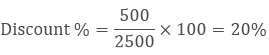Estimation in Percentages

To understand the estimation let’s take an example

Example

If the price of a product in the mall is 498.80 and it is available at a discount of 15 % then how would you estimate the amount to be paid?

Solution

These are the steps to estimate the percentage-

a. Round off the value 498.80 to the nearest tens i.e. 500.

b. Calculate the 10% of 500.i.e.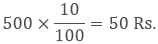c. Find the half of it i.e. 1/2 × 50 = 25.

d. Add both the values 50 + 25 = 75.

Therefore the bill amount will be reduced by approximately 75 Rs and you may have to pay 425 Rs.

Prices Related to Buying and Selling (Profit and Loss)

When we buy or sell anything in the market then there are so many things included in it like the cost of the product, some overhead expenses to present it, some promotion etc.

Terms Related to Buying and Selling

Cost Price (CP)

Cost price is the actual amount which is paid by the manufacturer to produce it or to provide the service.

Selling Price (SP)

It is the amount at which the product is sold in the market by the retailer.

Profit

After selling a product if the seller has some financial gains then it is said to be a profit.

Profit = SP – CPLoss

It is the financial negative revenue which a seller has to bear while selling the product.

Loss = CP – SP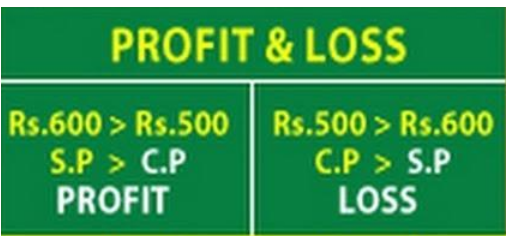Remark: While buying an item if a shopkeeper made some additional expense then these expenses are called Overhead Expenses. It will be included in the cost price.

Example

If a shopkeeper bought 250 books for 75 each. He spent 500 Rs. on the binding of books. Then he sold it in 20000 Rs. Calculate the profit or loss percentage.

Solution:

Price of 250 books = 250 × 75 = 18750 Rs.

Cost price of books = 18750 + 500 = 19250 Rs.

Sale Price = 20000 Rs.

SP > CP so he made a profit.

Profit = SP – CP

= 20000 – 19250

= 750 Rs.

His profit on 19250 Rs. is 750 Rs.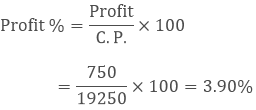Sales Tax/Value Added Tax/Goods and Services Tax

The amount charged by the government on the sale of an item is called Sales Tax.
Shopkeeper collects it from the customer and gives to the government. So the sales tax will always be on the selling price of the item and is added to the value of the bill.Earlier you must have seen Sales tax on the bill, nowadays; you will mostly see Value Added Tax

Formula

If the tax is x%, then Total price after including tax would be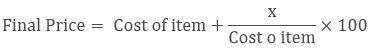Example

If the sales tax of 5% is added to an item whose selling price is 1500 Rs then find the bill amount including tax.

Solution:

The tax amount = 5/100 x 1500 = 75 Rs
Hence, the bill amount = Selling Price + Tax

= 1500 Rs + 75 Rs

= 1575 Rs.

Compounded Interest

Interest is the extra money paid by the banks on our money at a fixed rate of interest.Where P = Principal amount

R = Rate of interest

T = Time period for which the interest is paid

The interest calculated both on the principal and the interest earned is called compounded interest

Example

A sum of Rs. 20,000 is invested by Honey for 2 years at an interest of 8% compounded annually. Find the Compound Interest (C.I.) and the amount she has to pay at the end of 2 years.

Solution:

Step 1 Firstly calculate the Simple Interest (S.I.) for one year.

Let the principal for the first year be P1. Here, P1 = Rs.20, 000

SI1 = SI at 8% p.a. for 1st year = (20000 × 8)/100 = 1600 Rs.

Step 2: now find the principal for the next year

Amount at the end of 1st year = P1 + SI1 = Rs. 20000 + Rs. 1600 = Rs. 21600 = P2 (Principal for 2nd year)

Step 3: Now the new principal for another year = 21600 Rs.

SI2 = SI at 8% p.a. for 2nd yearAmount at the end of 2nd year = P2 + SI2 = Rs. 21600 + Rs.1728 = Rs. 23328

The Formula for Compound Interest

As the above method is very long and it cannot be used at every place so we can use the formula to find the compound interest.Compound Interest (CI) = Amount (A) – Principal (P)

Example

If a person borrow Rs 15,000 for 3 years at an interest rate of 10% compounded annually. Then what will be Compound Interest and the amount to be paid by him?

Solution:

Given,

P = 15000

r = 10%

n = 3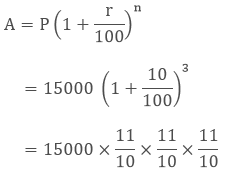= 19965 Rs.

CI = A – P

= 19965 - 15000

= 4965 Rs.

Rate Compounded Annually or Half Yearly (Semi-Annually)

The above formula is used when we have to find the interest compounded annually.

But sometimes it happens that we have to calculate the compound Interest half-yearly or quarterly.

In the case of half yearly, the time period will get double and the rate of interest will get half.

The Formula for the Rate Compounded Half Yearly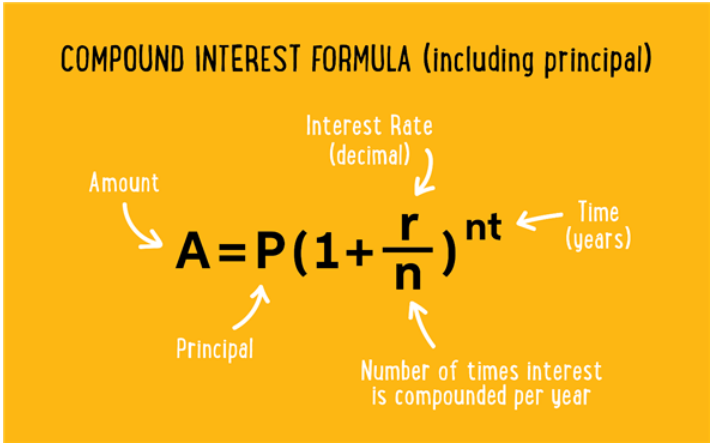Similarly, interest is calculated for compounded quarterly.

Applications of Compound Interest Formula

There are so many practical situations where the concept of compound interest is used.

The rate of growth of population.

The rate of growth of bacteria, etc.

```### Course Features

• 728 Video Lectures
• Revision Notes
• Previous Year Papers
• Mind Map
• Study Planner
• NCERT Solutions
• Discussion Forum
• Test paper with Video Solution